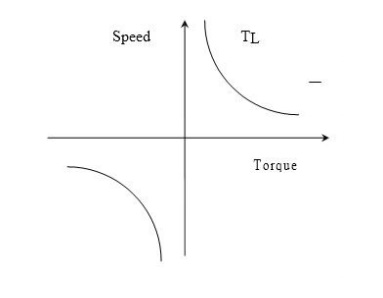Home | | Solid State Drives | Classification of Load Torques

## Chapter: Solid State Drives : Drive Characteristics

Load torques which has the potential to drive the motor under equilibrium conditions are called active load torques. Such load torques usually retain their sign when the drive rotation is changed (reversed)

Eg:

ü Torque due to force of gravity

ü Torque due tension

ü Torque due to compression and torsion etc

Load torques which always oppose the motion and change their sign on the reversal of motion are called passive load torques

Eg:

ü Torque due to friction, cutting etc.

The load torque T can be further divided in to following components

ü Friction Torque (T):

Friction will be present at the motor shaft and also in various parts of the load. T is the equivalent value of various friction torques referred to the motor shaft.

ü Windage Torque (T)

When motor runs, wind generates a torque opposing the motion. This is known as windage torque.

ü Torque required to do useful mechanical work

Nature of this torque depends upon particular application. It may be constant and independent of speed. It may be some function of speed, it may be time invariant or time variant, its nature may also change with the load’s mode of operation.

Friction at zero speed is called diction or static friction. In order to start the drive the motor should at least exceeds diction.

Friction torque can also be resolved into three componentsComponent Tvaries linearly with speed is called VISCOUS friction and is given by

Tv = B ωm

Where B is viscous friction co-efficient.

Another component T, which is independent of speed, is known as COULOMB friction. Third component Ts accounts for additional torque present at stand still. Since Tis present only at stand still it is not taken into account in the dynamic analysis. Wind age torque, T which is proportional to speed Squared is given byFrom the above discussions, for finite speed## Characteristics of Different types of Loads

One of the essential requirements in the section of a particular type of motor for driving a machine is the matching of speed-torque characteristics of the given drive unit and that of the motor. Therefore the knowledge of how the load torque varies with speed of the driven machine is necessary. Different types of loads exhibit different speed torque characteristics. However, most of the industrial loads can be classified into the following four categories.

ü Torque proportional to speed (Generator Type load)

ü Torque proportional to square of the speed (Fan type load)

ü Torque inversely proportional to speed (Constant power type load)

## Constant Torque characteristics:

Most of the working machines that have mechanical nature of work like shaping, cutting, grinding or shearing, require constant torque irrespective of speed. Similarly cranes during the hoisting and conveyors handling constant weight of material per unit time also exhibit this type of Characteristics

## Torque Proportional to speed:

Separately excited dc generators connected to a constant resistance load, eddy current brakes have speed torque characteristics given by

T=k## Torque proportional to square of the speed:

Another type of load met in practice is the one in which load torque is proportional to the square of the speed.

Examples:

ü Fans rotary pumps,

ü Compressors

ü Ship propellers## Torque Inversely proportional to speed:

Certain types of lathes, boring machines, milling machines, steel mill coiler and electric traction load exhibit hyperbolic speed-torque characteristicsStudy Material, Lecturing Notes, Assignment, Reference, Wiki description explanation, brief detail
Solid State Drives : Drive Characteristics : Classification of Load Torques |